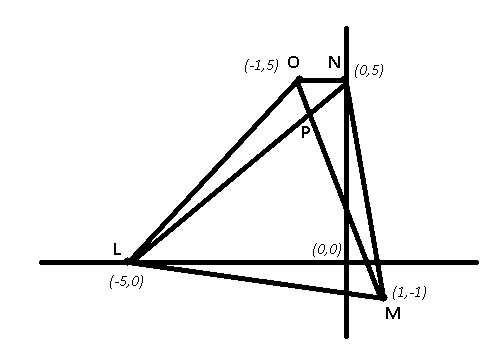Question 88

# ln a plane rectangular coordinate system, points L, M, N and O are represented by the coordinates (-5, 0), (1,-1), (0, 5), and (-1, 5) respectively. Consider a variable point P in the same plane. The minimum value of PL + PM + PN + PO is

Solution

$$(PL + PN)$$ will be minimum if P lies on LN, and $$(PM + PO)$$ will be minimum if P lies on OM.

=> P must be the intersection point of the diagonals of the quadrilateral.$$\therefore$$ Min (PL + PM + PN + PO)

= $$LN + OM$$

= $$(\sqrt{(0 + 5)^2 + (5 - 0)^2}) + (\sqrt{(1 + 1)^2 + (-1 - 5)^2})$$

= $$(\sqrt{25 + 25}) + (\sqrt{4 + 36})$$

= $$\sqrt{50} + \sqrt{40} = 5 \sqrt{2} + 2 \sqrt{10}$$